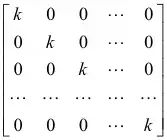## Filters

Q

Let A be a matrix such that   a scalar matrix and . Then A2 equals :

• Option 1)

• Option 2)

• Option 3)

• Option 4)

97 Views
N

As we learned

Scalar Matrix -

A diagonal matrix whose all the elements are equal is called a scalar matrix

- whereinis a scalar matrix

Let scalar matrix be

also

Take k = 6

Option 1)

Option 2)

Option 3)

Option 4)

Exams
Articles
Questions